# Dual axis charts in ggplot2 - why they can be useful and how to make them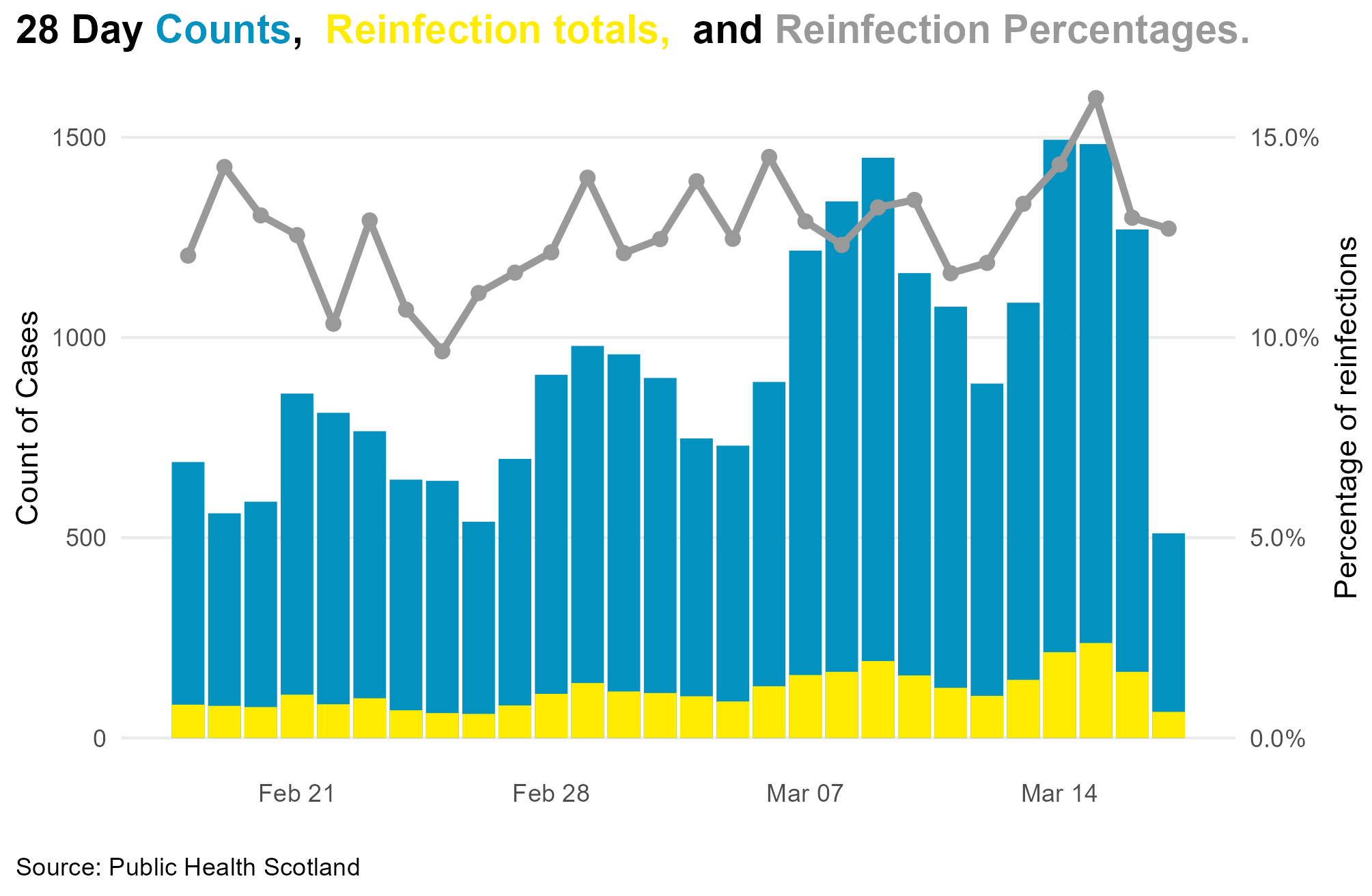Create Pareto charts in ggplot2 with custom legends using manual scales and ggtext

I had to produce a dual axis chart as part of a piece of work transitioning an Excel report to markdown.

I thought I’d do a walkthrough of a chart that is similar in style, but different in content than the one I produced at work.

I’m using COVID-19 open data from Public Health Scotland.

You can find this via the link in the code below, where you should find the latest daily data.

For work, I’ve been using rolling 7 day counts and rates per 100,000 population.

For this example, I’m just using the latest 28 days (at time of writing) and purely using counts, not rates. These are not rolling calculations, just straight counts direct from the data source.

library(data.table)
library(lubridate)
library(ggplot2)
library(ggExtra)

yellow <- "#FFEC00"   #NHS SCOTLAND YELLOW
lightblue <- "#0391BF"   # NHS SCOTLAND LIGHT BLUE


This next bit makes sure the URL updates each day so you are always looking for the latest data

# make the link dynamic
part1 <- "https://www.opendata.nhs.scot/dataset/"
part2 <- "b318bddf-a4dc-4262-971f-0ba329e09b87/"
part3 <- "resource/427f9a25-db22-4014-a3bc-893b68243055/"
part5 <- ".csv"
today <- gsub('-','',as.character(Sys.Date()))
link <- paste0(part1, part2, part3, part4, today, part5, sep = '')
dates <- seq.Date(as.Date(Sys.Date()-28), as.Date(Sys.Date() - 1), by = '1 day')
DT[, Date := lubridate::ymd(Date)]



(As a quick aside, I sometimes use data.table’s date functions for date manipulation.
I have also tried other date parsing packages, but have found that while they promise fast parsing, they regularly fail to recognise the correct date formats.
However, I should usually stick to lubridate - it’s great for parsing dates, and ymd(Date) is as good as it gets).

Now I’m just going to grab a subset of the data, and, for something different (to me at least) I’m filtering for data from Glasgow.

positives <- DT[Date >= as.Date(Sys.Date() -28) & CAName == 'Glasgow City',
.(Date, CAName, DailyPositive,
FirstInfections, Reinfections)] # grab a handful of relevant columns

#fwrite(positives, 'positives.csv') # in case you want to come back to the same data later

positives[,PercentReinfections := (Reinfections/DailyPositive)]



Although the PercentReinfections metric already exists in the data in a decimalised percent format, I’m recalculating it in it’s raw form to make the next bit easier.

## Step 1 - the basic plot

p1 <- ggplot(positives, aes(Date, DailyPositive, fill = 'DailyPositive')) +
geom_col() +
geom_col(aes(Date, Reinfections, fill = 'Reinfections')) +
theme_minimal() +
ggExtra::removeGrid() +
theme(legend.position = 'top',
plot.title.position = "plot",
plot.caption.position =  "plot",
legend.title = element_text(size = 11),
plot.title = element_text(hjust = 0.0),
plot.caption = element_text(hjust = 0),
axis.title.y.right = element_text( angle = 90))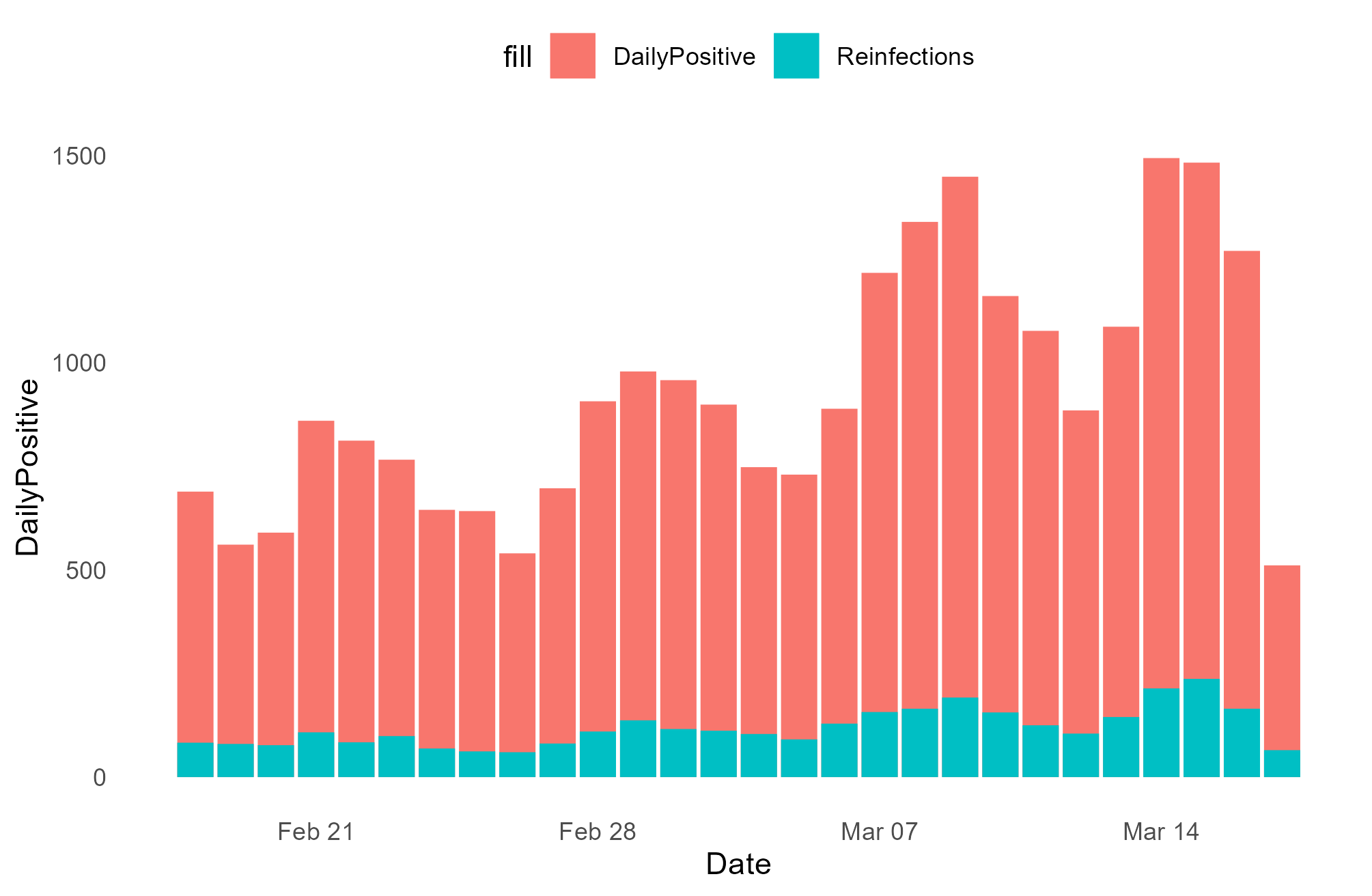## Add the dual axis

This needed a bit of jiggery-pokery to get the second axis on a reasonable scale.
If you haven’t done this before, you define that you want a secondary axis with the sec_axis argument to scale_y_continuous

You will need to transform it - here I am telling it to divide the value by 10,000. Again , you will need to play around to see what works for you.

p1 <- p1 +
geom_line(mapping = aes(Date, PercentReinfections * 10000),
colour = 'grey70', size = 1.2) +
geom_point(mapping = aes(Date, PercentReinfections * 10000),
colour = 'grey70', size = 2) +
scale_x_date(breaks = '2 days', date_labels = '%d %b %y') +
scale_y_continuous(sec.axis = ggplot2::sec_axis(~. / 10000,
name = "Percentage being reinfections",
labels = scales::label_percent()))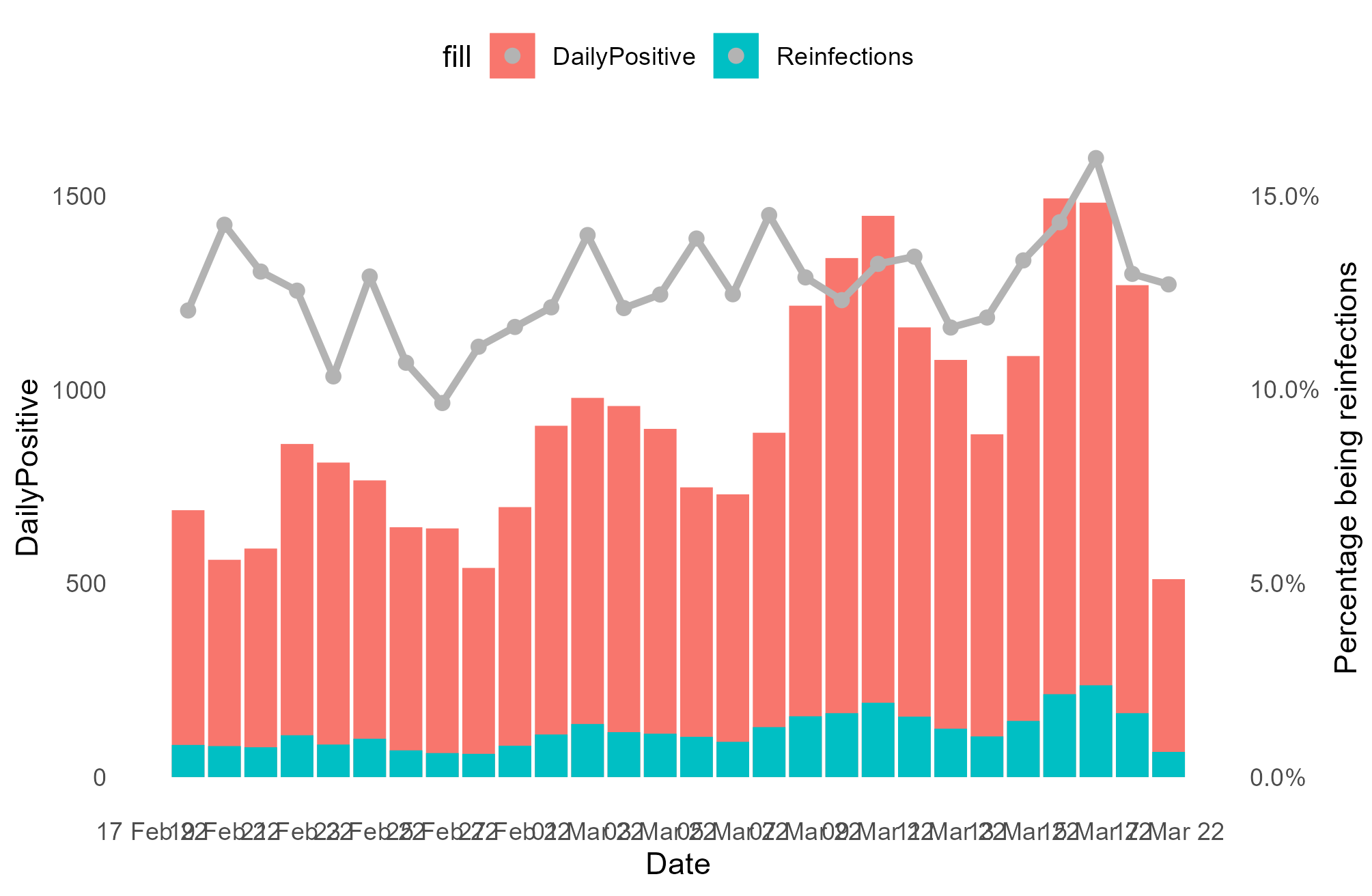This looks not too bad, but the legend is a mess. Time for a manual scale, with our chosen colours from above. I set the background for PercentReinfections to white so that I can keep the grey dot, while maintaining a solid fill for the other 2 measures

p1 <- p1 +
scale_fill_manual(name = NULL,
guide = "legend",
values = c("DailyPositive" = lightblue,
"Reinfections" = yellow,
"PercentReinfections" = "white"),
labels = c('Daily cases',
'Daily reinfections',
'Percent of reinfections'))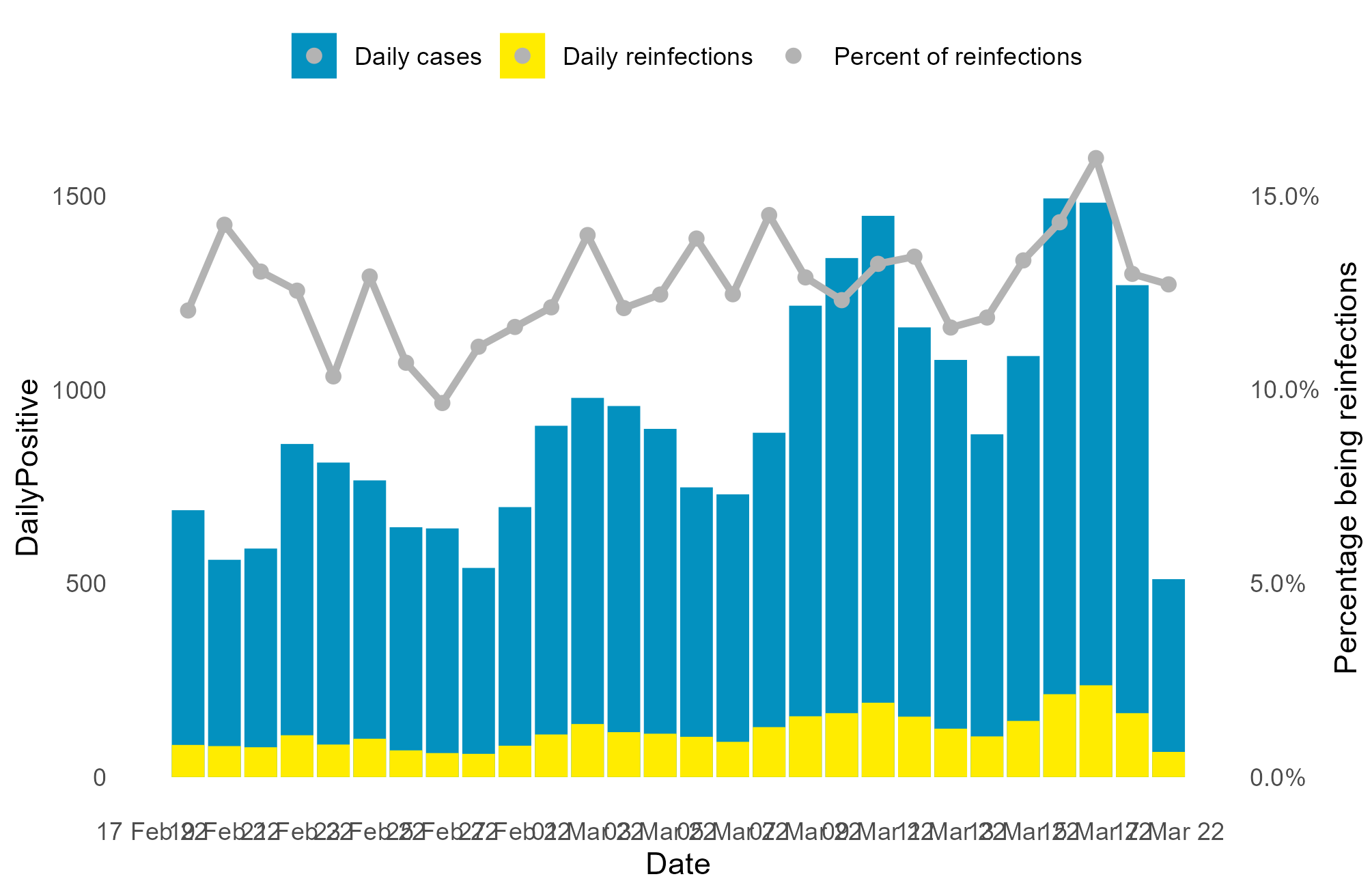Not too bad - but I don’t want the grey dot on top of the 2 blue shades.

Here’s how to get rid of those, while also adding in some labels:


p1 <- p1 +
guides(fill = guide_legend(override.aes = list(linetype = c(0, 0, 1),
shape = c(NA, NA, 16)
)))

p1 <- p1 +  labs(x = "Date",
y = " Count of cases",
caption = 'Source: Public Health Scotland',
title = "28 Day Cases by episode and reinfection rate") +
ggExtra::rotateTextX()

print(p1)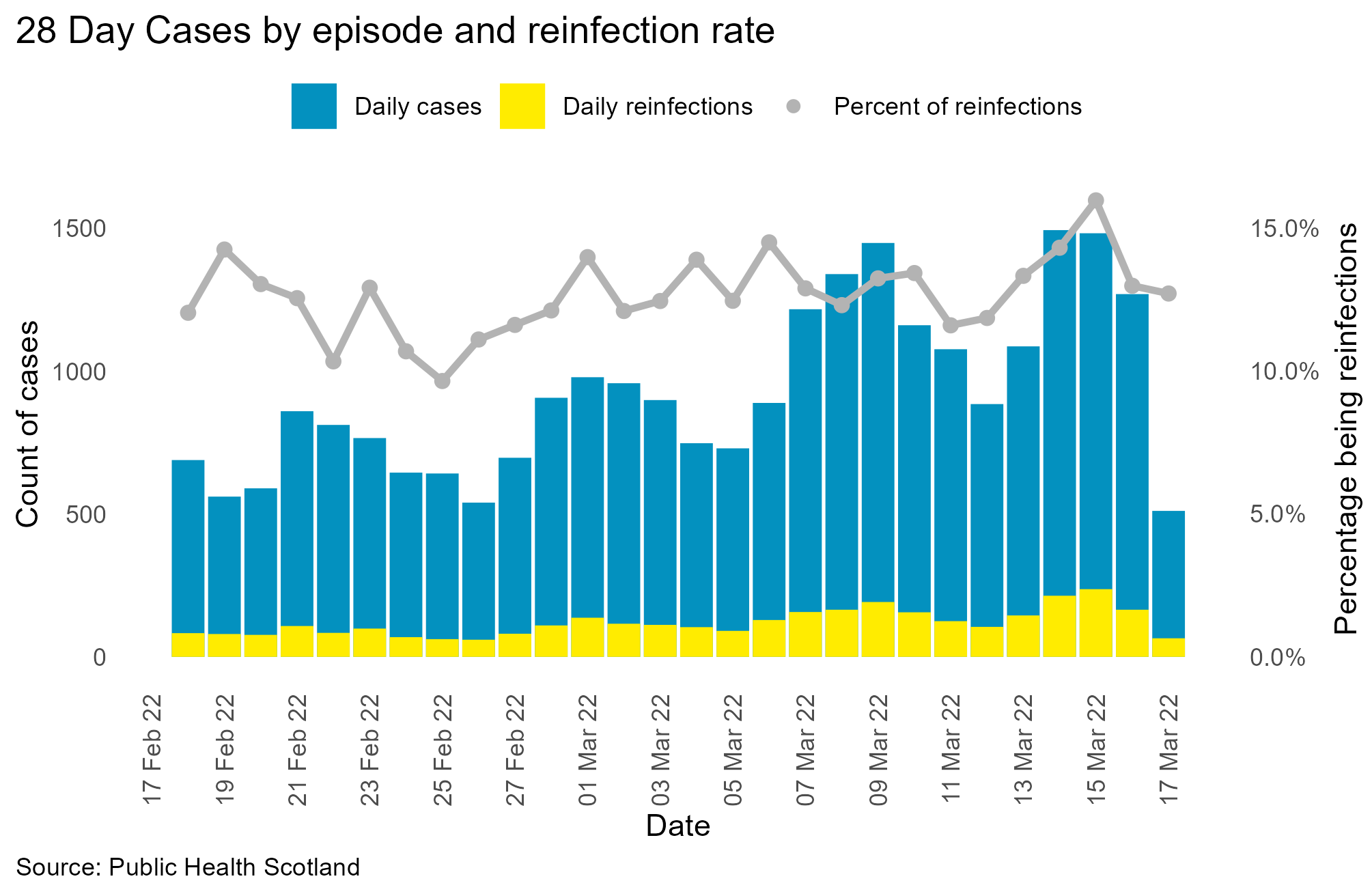Job done.

Here is another way using ggtext for coloured plot title, negating the need for a custom legend.
This means using some html, which, is oddly satisfying, if only for a few fleeting moments.

## Alternate with ggtext

p2 <-  ggplot(positives, aes(Date, DailyPositive)) +
geom_col(fill = lightblue) +
geom_col(aes(Date, Reinfections), fill = darkblue) +
theme_minimal() +
theme(legend.position = "top") +
ggExtra::removeGridX() +
labs(x = "",
y = "Count of Cases",
caption = 'Source: Public Health Scotland',
title = "<b>28 Day <span style = 'color:#0391BF;'>Counts</span>,
<span style = 'color:#FFEC00;'> Reinfection totals, </span> and
<span style = 'color:#999999;'>Reinfection Percentages.</span></b>") +
theme(
plot.title = element_textbox_simple(
size = 14,
lineheight = 1,
padding = margin(0, 0, 5, 0)),
legend.position = 'top',
plot.title.position = "plot",
plot.caption.position =  "plot",
legend.title = element_text(size = 11),
plot.caption = element_text(hjust = 0),
axis.title.y.right = element_text( angle = 90))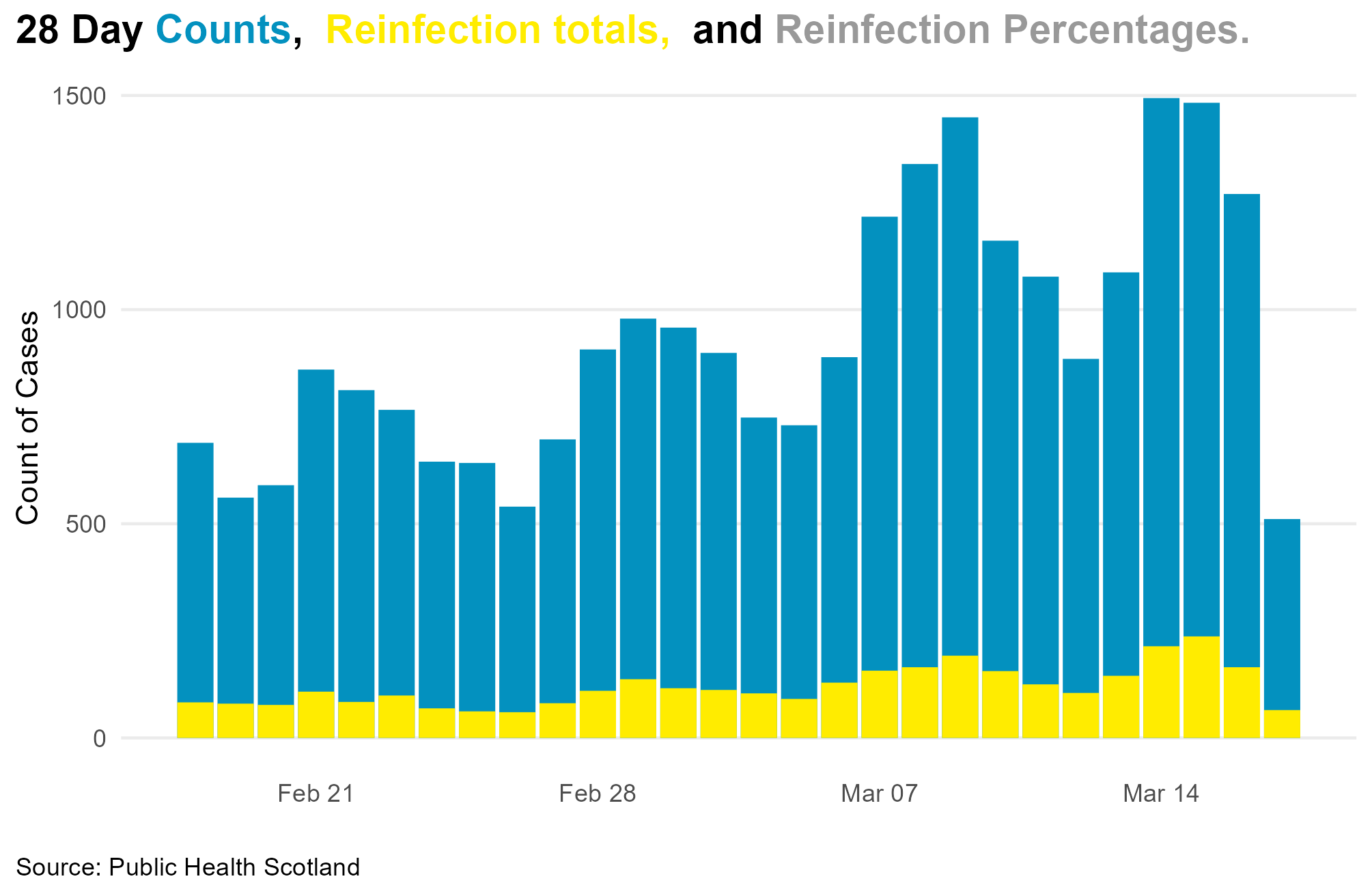Now to add the secondary axis and values

p2 <- p2 +
geom_line(mapping = aes(Date, PercentReinfections * 10000),
colour = 'grey60', size = 1.2) +
geom_point(mapping = aes(Date, PercentReinfections * 10000),
colour = 'grey60', size = 2) +
scale_y_continuous(sec.axis = ggplot2::sec_axis(~. / 10000,
name = "Percentage of reinfections",
labels = scales::percent))

print(p2)## Pareto Charts - a justifiable case?

A good use for dual axis charts (possibly the only really good one) is for Pareto charts.

A pareto chart combines both the count of an item, and the percentage contribution that count makes to the overall tally.

We’ve used Pareto Analysis to home in on wards where falls were particularly high - it may be, for example, that 5 wards, representing less than 20% of your available wards, are responsible for a high percentage of your overall falls. By concentrating improvement efforts on them, you can make huge reductions in overall falls rates.

In this next contrived example, I’m listing some fictitious contributory factors for patient falls - a fall can have more than one factor assigned to it.

In the first section, I order the data by descending order and pull out the contributory reasons as a vector, which I can then assign as an ordered factor, so the plot remains in descending order.

library(tibble)

fall_reasons <- tribble(
~contributory, ~count,
"Dementia",  40,
"Confusion", 30,
"Footwear",  10,
"Dizziness", 5,
"Walking aids", 3,
"Environment", 20
)

contrib_levels <- fall_reasons %>%
arrange(desc(count)) %>%
pull(contributory)

fall_reasons$contributory <- factor(fall_reasons$contributory,
ordered = TRUE,
levels = contrib_levels)

fall_reasons <- fall_reasons %>%
arrange(desc(count)) %>%
mutate(cum_percent = cumsum(count) / sum(count))

p3 <- ggplot(fall_reasons, aes(contributory, count)) +
geom_col(fill = yellow) +
theme_minimal() +
ggExtra::removeGrid() +
theme(legend.position = 'top',
plot.title.position = "plot",
plot.caption.position =  "plot",
legend.title = element_text(size = 11),
plot.title = element_text(hjust = 0.0),
plot.caption = element_text(hjust = 0),
axis.title.y.right = element_text( angle = 90))

p3 <- p3 + geom_hline(yintercept = 80, colour = 'grey80')

p3 <- p3 + geom_line(mapping = aes(contributory, cum_percent * 100, group = 1),
colour = lightblue, size = 1.2) +
geom_point(mapping = aes(contributory, cum_percent * 100), colour = lightblue, size = 2) +
scale_y_continuous(sec.axis = ggplot2::sec_axis(~. /100,
name = "Percent incidents where reason mentioned",
labels = scales::label_percent())) +
theme(legend.position = 'none')

p3 <- p3 +

labs(x = "Contributory Factor",
y = " Count of cases",
caption = 'Source: ENTIRELY MADE UP',
subtitle = 'Count, and percent contribution to total',
title = "Contributory factors in patient falls - Pareto Analysis")

p3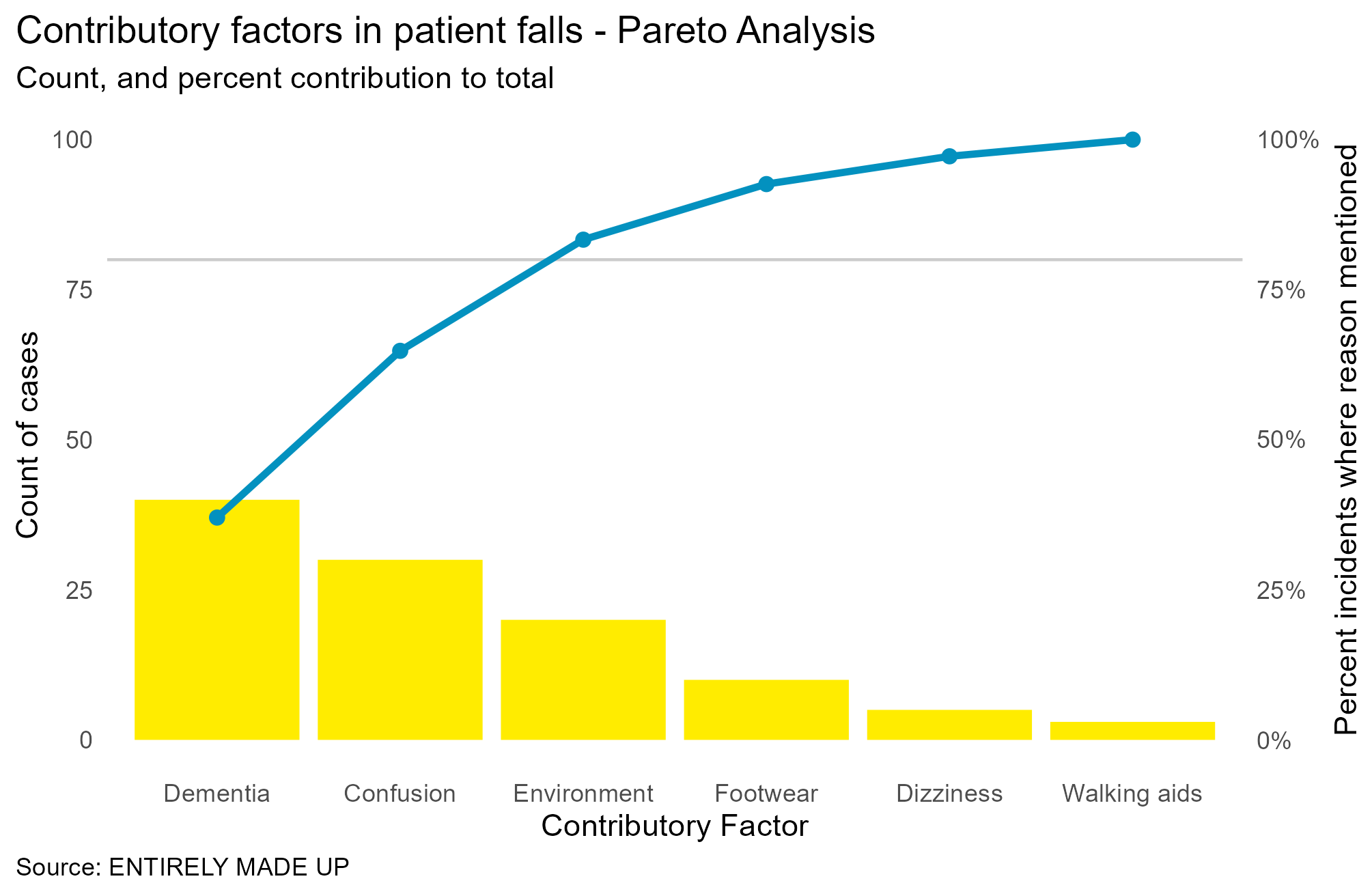By adding the horizontal line at the 80% mark, we can see where the cutoff is for the contributory factors - the first 3 account for the bulk of the results, and so if we work on addressing these, then we might see further reductions in falls.

This is a very basic example, but if you have not used Pareto charts, and you want to drill down into the causes of issues, then they are very handy tools.
They are also easily understood by non-data people - so you don’t need to get bogged down in statistics, if you’d rather keep things simple.